### Home > INT1 > Chapter Ch8 > Lesson 8.1.1 > Problem8-9

8-9.
1. Solve each equation for the indicated variable. Homework Help ✎

1. y = 3x + 2 (for x)

2. a =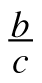(for b)

3.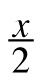7 =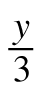(for x)

4. g =at2 (for t2)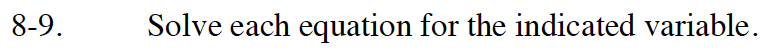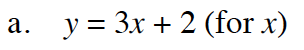Isolate the term with the x on one side of the equation.

$x=\frac{y-2}{3}$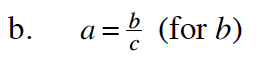Multiply both sides by c
(Multiplication Property of Equality).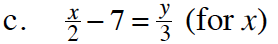$x=\frac{2y}{3}+14$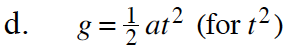$t^2=\frac{2g}{a}$